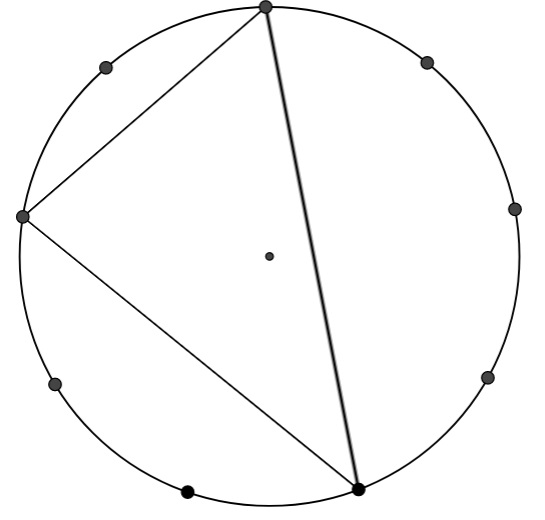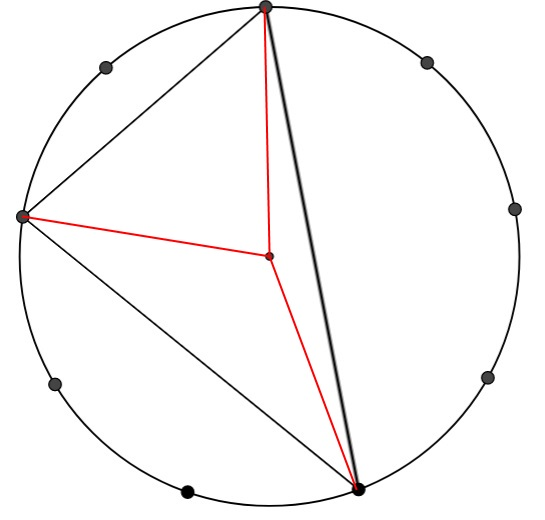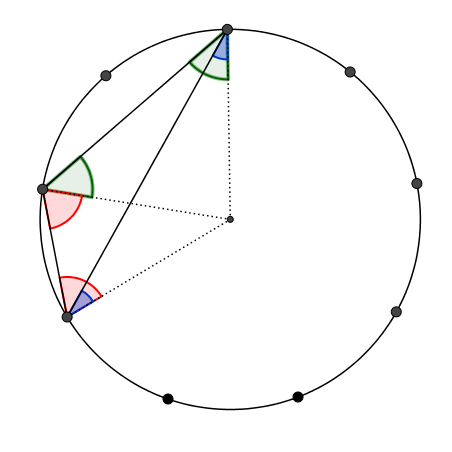#### You may also like### Coins on a Plate

Points A, B and C are the centres of three circles, each one of which touches the other two. Prove that the perimeter of the triangle ABC is equal to the diameter of the largest circle.### Not So Little X

Two circles are enclosed by a rectangle 12 units by x units. The distance between the centres of the two circles is x/3 units. How big is x?### The Cyclic Quadrilateral

This gives a short summary of the properties and theorems of cyclic quadrilaterals and links to some practical examples to be found elsewhere on the site.

# Triangles in Circles

##### Age 11 to 14Challenge Level
If you prefer to work on paper, you may wish to print out some 9 dot circles

In the GeoGebra interactivity below there is a circle with 9 equally spaced points on the edge, and one in the centre.

Draw as many different triangles as you can, by joining the centre dot and any two of the dots on the edge.

Can you work out the angles in your triangles?

You should have found four different triangles with angles of:
40, 70, 70
80, 50, 50
120, 30, 30,
160, 10, 10

Here is a triangle formed by joining three dots on the edge of the nine-point circle:Can you work out the angles of this triangle?
Click to reveal a diagram that might help you work out the angles.Create some more triangles by joining three dots on the edge of the nine point circle.
How many different triangles can you make?
Can you work out the angles each time?

When the centre dot isn't inside your triangle, you might find it a little trickier to work out the angles. Click below for a diagram that might help:Many thanks to Geoff Faux who introduced us to the merits of the 9 pin circular geo-board.